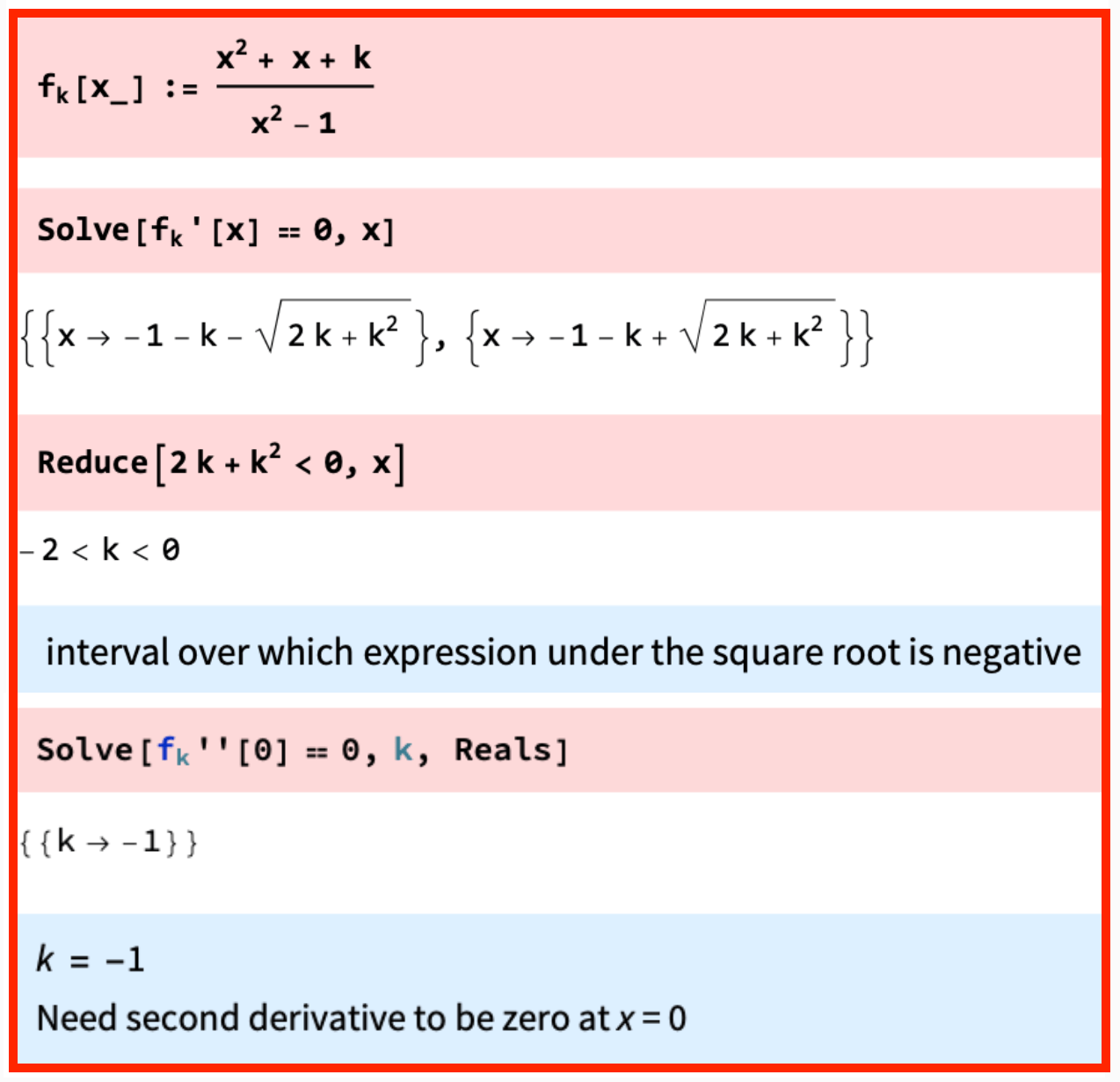## WitCH 38: A Deep Hole

This one is due to commenter P.N., who raised it on another post, and the glaring issue has been discussed there. Still, for the record it should be WitCHed, and we’ve also decided to expand the WitCHiness slightly (and could have expanded it further).

The following questions appeared on 2019 Specialist Mathematics NHT, Exam 2 (CAS). The questions are followed by sample Mathematica solutions (screenshot corrected, to include final comment) provided by VCAA (presumably in the main for VCE students doing the Mathematica version of Methods). The examination report provides answers, identical to those in the Mathematica solutions, but indicates nothing further.## UPDATE (05/07/20)

The obvious problem here, of course, is that the answer for Part (b), in both the examination report and VCAA’s Mathematica solutions, is flat out wrong: the function fk will also fail to have a stationary point if k = -2 or k = 0. Nearly as bad, and plenty bad, the method in VCAA’s Mathematica solutions to Part (c) is fundamentally incomplete: for a (twice-differentiable) function f to have an inflection point at some a, it is necessary but not sufficient to have f’’(a) = 0.

That’s all pretty awful, but we believe there is worse here. The question is, how did the VCAA get it wrong? Errors can always occur, but why specifically did the error in Part (b) occur, and why, for a year and counting, wasn’t it caught? Why was a half-method suggested for Part (c), and why was this half-method presumably considered reasonable strategy for the exam? Partly, the explanation can go down to this being a question from NHT, about which, as far as we can tell, no one really gives a stuff. This VCAA screw-up, however, points to a deeper, systemic and much more important issue.

The first thing to note is that Mathematica got it wrong: the Solve function did not return the solution to the equation fk‘ = 0. What does that imply for using Mathematica and other CAS software? It implies the user should be aware that the machine is not necessarily doing what the user might reasonably think it is doing. Which is a very, very stupid property of a black box: if Solve doesn’t mean “solve”, then what the hell does it mean? Now, as it happens, Mathematica’s/VCAA’s screw-up could have been avoided by using the function Reduce instead of Solve.* That would have saved VCAA’s solutions from being wrong, but not from being garbage.

Ask yourself, what is missing from VCAA’s solutions? Yes, yes, correct answers, but what else? This is it: there are no functions. There are no equations. There is nothing, nothing at all but an unreliable black box. Here we have a question about the derivatives of a function, but nowhere are those derivatives computed, displayed or contemplated in even the smallest sense.

For the NHT problem above, the massive elephant not in the room is an expression for the derivative function:What do you see? Yep, if your algebraic sense hasn’t been totally destroyed by CAS, you see immediately that the values k = 0 and k = -2 are special, and that special behaviour is likely to occur. You’re aware of the function, alert to its properties, and you’re led back to the simplification of fk for these special values. Then, either way or both, you are much, much less likely to screw up in the way the VCAA did.

And that always happens. A mathematician always gets a sense of solutions not just from the solution values, but also from the structure of the equations being solved. And all of this is invisible, is impossible, all of it is obliterated by VCAA’s nuclear weapon approach.

And that is insane. To expect, to effectively demand that students “solve” equations without ever seeing those equations, without an iota of concern for what the equations look like, what the equations might tell us, is mathematical and pedagogical insanity.

*) Thanks to our ex-student and friend and colleague Sai for explaining some of Mathematica’s subtleties. Readers will be learning more about Sai in the very near future.